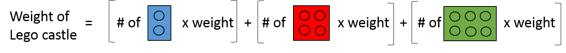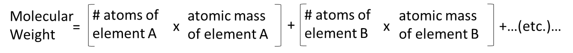Molecular Weight: Definition & Formula

Instructor: Carolyn George
In this lesson, we will learn about molecular weight, which is the mass of a molecule determined by summing the masses of all of the atoms that make it up. We will look at the formula for determining molecular weight, learn where to find atomic masses on the periodic table, and do an example calculation.

What is Molecular Weight?

Molecular weight is the weight of a molecule. Actually, the term weight is a little misleading since molecular weights are measured in units of mass, which are not weights at all. For this reason, molecular weight is often more accurately called molecular mass or formula mass.

How Do You Calculate It?

Imagine you made a castle out of Legos and you want to know how much it weighs. You could just put it on a scale and weigh it. But what if the castle was too big to fit on the scale? How could you determine how much it weighs without taking it apart? One thing that you could do is count how many Legos were used to make the castle. Then you could determine the average weight of a Lego by weighing some Legos individually. Multiply the number of Legos in the castle by their average weight and you have the weight of your castle.

But wait! Did you say that not all the Legos used were the same size? That's right. Instead, you would have to count each type of Lego in the castle, multiply by the average weight of each type, and then add them all together.Now, think of your Lego castle as a molecule and the individual Legos are the pieces that make up the molecule, the atoms. Just like the Lego castle was too big to weigh on a scale, so too are molecules too small to weigh on a scale (at least not individually). And just like Legos in a castle come in different sizes and are present in different amounts, so too do atoms come in different sizes and are present in different amounts within a molecule. So, we just have to know how many of each type of atom are in a molecule and the average mass of each so we can calculate the molecular weight.

The formula for determining molecular weight is:To unlock this lesson you must be a Study.com Member.

Register to view this lesson

Are you a student or a teacher?